## Kobe is a basketball player. He is able to make a free throw 70% of the time. What is the probability that Kobe makes his 10th free throw on

Question

Kobe is a basketball player. He is able to make a free throw 70% of the time. What is the probability that Kobe makes his 10th free throw on his 14th shot

in progress 0
3 months 2021-08-03T17:54:47+00:00 1 Answers 4 views 0

0.1636 = 16.36% probability that Kobe makes his 10th free throw on his 14th shot

Step-by-step explanation:

For each free throw, there are only two possible outcomes. Either he makes it, or he misses. The probability of making a free throw is independent of any other free throw, which means that the binomial probability distribution is used to solve this question.

Binomial probability distribution

The binomial probability is the probability of exactly x successes on n repeated trials, and X can only have two outcomes.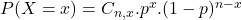In which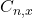is the number of different combinations of x objects from a set of n elements, given by the following formula.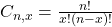And p is the probability of X happening.

He is able to make a free throw 70% of the time.

This means that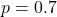What is the probability that Kobe makes his 10th free throw on his 14th shot?

9 of his first 13(P(X = 9) when n = 13), and then the 10th with 0.7 probability.

Thus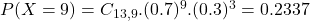0.7*0.2337 = 0.1636

0.1636 = 16.36% probability that Kobe makes his 10th free throw on his 14th shot